Next: Motion in a General Up: Planetary Motion Previous: Orbital Energies

Kepler Problem

In a nutshell, the so-called Kepler problem consists of determining the radial and angular coordinates,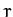and, respectively, of an object in a Keplerian orbit about the Sun as a function of time.

Consider an object in a general Keplerian orbit about the Sun which passes through its perihelion point,and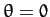, at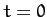. It follows from the previous analysis that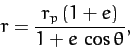(276)

and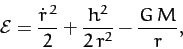(277)

where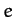,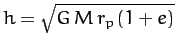, and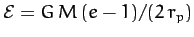are the orbital eccentricity, angular momentum per unit mass, and energy per unit mass, respectively. The above equation can be rearranged to give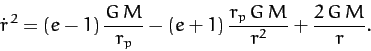(278)

Taking the square-root, and integrating, we obtain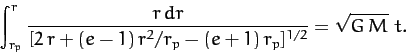(279)

Consider an elliptical orbit characterized by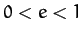. Let us write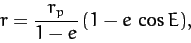(280)

whereis termed the elliptic anomaly. In fact,is an angle which varies between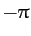and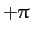. Moreover, the perihelion point corresponds to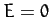, and the aphelion point to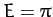. Now,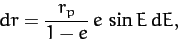(281)

whereas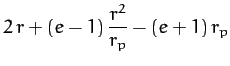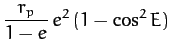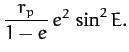(282)

Thus, Equation (279) reduces to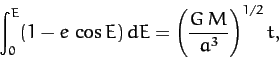(283)

where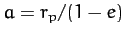. This equation can immediately be integrated to give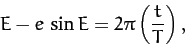(284)

where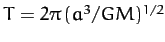is the orbital period. Equation (284), which is known as Kepler's equation, is a transcendental equation which does not possess a simple analytic solution. Fortunately, it is fairly straightforward to solve numerically. For instance, using an iterative approach, if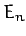is theth guess then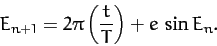(285)

The above iteration scheme converges very rapidly (except in the limit as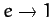).

Equations (276) and (280) can be combined to give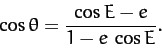(286)

Thus,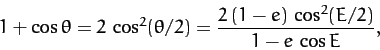(287)

and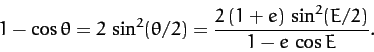(288)

The previous two equations imply that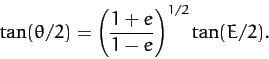(289)

We conclude that, in the case of an elliptical orbit, the solution of the Kepler problem reduces to the solution of the following three equations: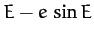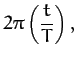(290)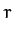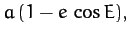(291)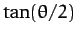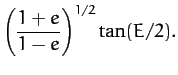(292)

Here,and. Incidentally, it is clear that if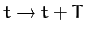then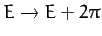, and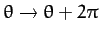. In other words, the motion is periodic with period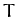.

For the case of a parabolic orbit, characterized by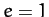, similar analysis to the above yields: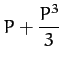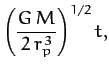(293)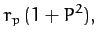(294)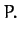(295)

Here,is termed the parabolic anomaly, and varies between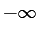and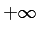, with the perihelion point corresponding to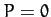. Note that Equation (293) is a cubic equation, possessing a single real root, which can, in principle, be solved analytically. However, a numerical solution is generally more convenient.

Finally, for the case of a hyperbolic orbit, characterized by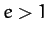, we obtain: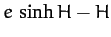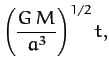(296)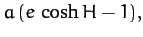(297)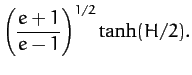(298)

Here,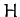is termed the hyperbolic anomaly, and varies betweenand, with the perihelion point corresponding to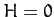. Moreover,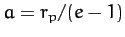. As in the elliptical case, Equation (296) is a transcendental equation which is most easily solved numerically.Next: Motion in a General Up: Planetary Motion Previous: Orbital Energies
Richard Fitzpatrick 2011-03-31